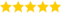# How to Input a List in Python?

Last updated on 25th Sep 2020, Artciles, Blog

Dheena (Sr Technical Project Manager - Python )

He is Highly Experienced in Respective Technical Domain with 11+ Years, Also He is a Respective Technical Trainer for Past 5 Years & Share's This Important Articles For us.(5.0) | 13465 Ratings 491

Sometimes while coding in Python, you will need to make a list as an input. While this might sound simple at first, it is often regarded as a complex task to accomplish for a beginner.

### Input a List in Python

As you might already know, in order to accept input from the user in Python, we can make use of the input() function. When used, it enables the programmer to accept either a string, integer, or even a character as an input from the user. But when it comes to accepting a list as an input, the approach we follow is slightly different.

### Example

• input_string = input(“Enter a list element separated by space “)
• list  = input_string.split()
• print(“Calculating sum of element of input list”)
• sum = 0
• for num in list:
• sum += int (num)
• print(“Sum = “,sum)

### Output

• Enter a list element separated by space 2 4 6 9
• Calculating sum of element of input list
• Sum = 20

### Now, let us understand how this program works:

1. 1.As you already know, whenever we use the input() function in Python, it converts the user input into a string. Therefore in the above program, we accepted a list element from a user in the form of a string that is separated by spaces.
2. 2.One thing to note here is that you have the ability to accept a string separated by the operator comma (,) as well. But in this situation, you need to make use of the split() function in order to pass the argument as well as the separator in the Python program.
3. 3.If you look closely, you will find that we have made use of the function input_string.split() to split the input string separated by spaces from the user and converted them into individual elements to be added into a list.
4. 4.We have also made use of the For loop and converted every element into an integer to calculate its sum.

### Example

• input_string = input(“Enter family members separated by comma “)
• family_list  = input_string.split(“,”)
• print(“Printing all family member names”)
• for name in family_list:
• print(name)

### Output

• Enter family members separated by a comma: Arvindh,Nivaas
• Printing all family member names
• Arvindh
• Gaviya

### Now, let us understand how this program works:

1. 1.Similar to the earlier example, we accepted an input list from the user in the form of a string separated by commas.
2. 2.We have made use of the input_string.split(“,”) function to split the string separated by commas and convert it into a list of strings to be used in the program.
3. 3.We used a for loop and printed out all family names in order, as you can see in the output shared above.

### With format and input

The format function can be used to fill in the values in the place holders and the input function will capture the value entered by the user. Finally, we will append the elements into the list one by one.

### Example

• listA = []
• # Input number of elemetns
• n = int(input(“Enter number of elements in the list : “))
• # iterating till the range
• for i in range(0, n):
• print(“Enter element No-{}: “.format(i+1))
• elm = int(input())
• listA.append(elm) # adding the element
• print(“The entered list is: \n”,listA)

### Output

• Running the above code gives us the following result −
• Enter number of elements in the list : 4
• Enter element No-1:
• 7
• Enter element No-2:
• 45
• Enter element No-3:
• 1
• Enter element No-4:
• 74
• The entered list is:
• [7, 45, 1, 74]

### With map

Another approach is to ask the user to enter the values continuously but separated by comma. Here we use the map function together the inputs into a list.

### Example

• listA = []
• # Input number of elemetns
• n = int(input(“Enter number of elements in the list : “))
• # Enter elements separated by comma
• listA = list(map(int,input(“Enter the numbers : “).strip().split(‘,’)))[:n]
• print(“The entered list is: \n”,listA)

### Output

• Running the above code gives us the following result −
• Enter number of elements in the list : 4
• Enter the numbers : 12,45,65,32
• The entered list is:
• [12, 45, 65, 32]

### Entering list of lists

We can also use input function twice so that we can create a list of lists. Use the range function to keep account on number of elements to be entered and the format function to enter the elements one by one. Finally, we append each entered element to the newly created list.

### Example

• listA = []
• # Input number of elemetns
• n = int(input(“Enter number of elements in the list : “))
• # Each sublist has two elements
• for i in range(0, n):
• print(“Enter element No-{}: “.format(i + 1))
• ele = [input(), int(input())]
• listA.append(ele)
• print(“The entered list is: \n”,listA)

### Output

• Running the above code gives us the following result −
• Enter number of elements in the list : 2
• Enter element No-1:
• ‘Mon’
• 3
• Enter element No-2:
• ‘Tue’
• 4
• The entered list is:
• [[“‘Mon'”, 3], [“‘Tue'”, 4]]

Are you looking training with Right Jobs?